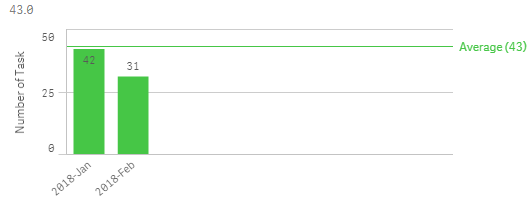# New to Qlik Sense

Discussion board where members can get started with Qlik Sense.

Contributor

## Subtitle expression.

Hi. May I know why my number of task expression show different value when used in subtitle expression? What is the correct expression?

It show 43. what i want is 36.5

• 42+31=73
• 73/2= 36.5

Expression for number of task:

=Num(Count({\$<[SR No]={"=Min ({<Temp = {'Task_1'}>}[Date Created]) >  MakeDate(2018,1,15)"}>

([SR No])),'##.0')